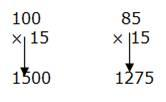## Percentage For SBI PO : Set – 04

1) A shopkeeper sells books at the rate of Rs. 455 each and earns a commission of 4%. He also sells pen boxes at the rate of Rs.75 each and earns a commission of 20%. How much amount of commission will he earn in two weeks if he sells 20 books and 12 pen boxes a day?

a) Rs. 7,956

b) Rs. 8,586

c) Rs. 6,716

d) Rs. 7,696

e) None of these

e)

Total number of books sold in 2 weeks=> 2 × 7 × 20 = 280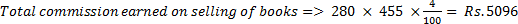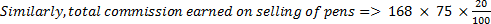= Rs. 2520

Total commission earned = Rs.5096 + Rs.2520

= Rs.7616

2) In a test, minimum passing percentage for boys and girls is 45% and 40% respectively. A girl scored 534 marks and failed by 98 marks. What is the minimum passing marks for boys?

a) 425

b) 625

c) 632

d) 711

e) None of these

d)

40% of minimum passing marks for girls = 534 + 98 => 632

40% => 632

100% => 1580

Minimum passing marks for boys,

= 1580 * 45/100

=711

3) Twelve per cent of vipin’s monthly salary is equal to sixteen per cent of Ravi’s monthly salary. Rahul’s monthly salary is half that of Ravi’s monthly salary. If Rahul’s annual salary is Rs 1.44 lacs, what is vipin’s monthly salary?

a) Rs. 48,000

b) Rs. 32,000

c) Rs. 40,000

d) Rs. 24,000

e) None of these

b)

Vibin’s salary         = x

Rahul’s salary        = z

Ravi’s salary          = y

12% of x = 16% y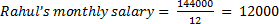Ravi’s monthly salary      = 2 × 12000

= 24000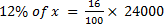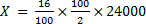X = 32000

4) If a person saves 25% of his monthly income and his income is increased by 14% and expenditure is increased by 15%. Then how much percentage would percentage of savings increase?

a) 25

b) 11

c) 23

d) 17

e) None of these

b)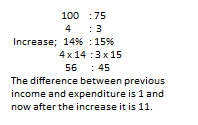5) A candidates scores 15% and fails by 30 marks, while another candidates who scores 40% marks, gets 20 marks more than then minimum required marks to pass the examination. Fine the maximum marks of the examination.

a) 200

b) 300

c) 150

d) 100

e) None of these

a)

Maximum marks = x

Pass marks = y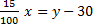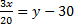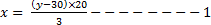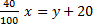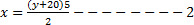From 1, 2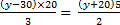2 (4y – 120) = 3y + 60

8y – 240 = 3y + 60

5y = 300

Y = 60

From – 2

=200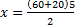=200

6) Raman scored 456 marks in an exam and Seeta got 54% marks in the same exam which is 24 marks less than Raman. If the minimum passing marks in the examination is 34%, then how much more marks did Raman score than the minimum passing marks?

a) 184

b) 196

c) 190

d) 180

e) None of these

a)

If total maximum marks be x than,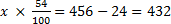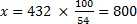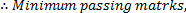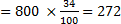= 456 – 272 = 184

7) The price of rice has increased by 60%. In order to restore the original price, the new price must be reduced by

a) 33.33%

b) 37.5%

c) 45%

d) 48%

e) None of these

b)

Reduced % = 100*60/100+60 = 100*60/160

8) Population of a district is 2,96,000 of which 1,66,000 are males. 50% of the population is literate. If 70% males are literate, then the number of women who are literate then the number of women who are literate is?

a) 32,900

b) 31,800

c) 35,295

d) 30,945

e) None of these

b)

Population of literate total =(50/100)*296000 = 1,48,000

Population of male literate = (70/100)*1,66,000

=1, 16, 200

Number of literate women = 1, 48, 000-1,16,200

=31,800

9) The discount series 10%, 20%, 40% is equivalent to a single discount of

a) 50%

b) 56.8%

c) 60%

d) 62.28%

e) None of these

b)

discount of 10% 20% 40% is equivalent to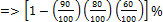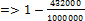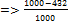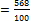10) A Bookseller allowed 15% discount on the books sold. Sunil purchased books worth Rs.1500. How much will he have to pay to Bookseller.

a) Rs.1200

b) Rs.1250

c) Rs.1275

d) Rs.1300

e) None of these